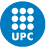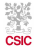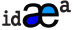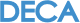Physics-informed Neural Networks to Simulate Subsurface Fluid Flow in Fractured Reservoirs.

Autor: Linus Walter (IMEDEA-CSIC, IDAEA-CSIC)

Physics-informed Neural Networks to Simulate Subsurface Fluid Flow in Fractured Reservoirs.

Abstract:

Modeling fluid flow in fractured media is computationally demanding and presents challenges related to the representation of the fracture network. In this study, we utilize state-of-the-art Physics-Informed Neural Networks (PINN) to simulate subsurface flow in fractured reservoirs. A PINN can incorporate any physical laws that can be described by partial differential equations. We obtain a ground truth dataset by running a virtual pumping-well test in the numerical code “OpenGeoSys”. This model consists of a low-permeability rock matrix, intersected by a high-permeability fracture. First, we approximate the reservoir permeability k(x) with an Artificial Neural Network (ANN). Second, we model the fluid pressure evolution p(x,t) with the PINN by informing it about the experimental well-testing data. Since observation wells are sparse in space (only the injection well in our case), we also ensure accordance of p(x) by satisfying mass conservation. The residual of this equation enforces the loss function of p(x,t) for random collocation points inside the domain. Our results indicate that the ANN is able to approximate k(x) even for a high permeability contrast. In addition, the successful interpolation of p(x,t) proves the PINN is a promising method for matching field data with physical laws.Mapa de sitio
Suscríbite